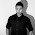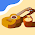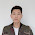Tuesday, November 25, 2014

[LeetCode] Permutation Sequence

The set [1,2,3,…,n] contains a total of n! unique permutations.
By listing and labeling all of the permutations in order,
We get the following sequence (ie, for n = 3):
1. "123"
2. "132"
3. "213"
4. "231"
5. "312"
6. "321"
Given n and k, return the kth permutation sequence.
Note: Given n will be between 1 and 9 inclusive.

1234
1243
1324
1342
1423
1432
2134
2143
2314  <= k = 9
2341
2413
2431
3124
3142
3214
3241
3412
3421
4123
4132
4213
4231
4312
4321

 1 2 3 4 5 6 7 8 9 10 11 12 13 14 15 16 17 18 19 20 21 22 23 24 class Solution { public: string getPermutation(int n, int k) { string ret; vector factorial(n,1); vector num(n,1); for(int i=1; i=1; i--) { int j = k/factorial[i-1]; k %= factorial[i-1]; ret.push_back(num[j]); num.erase(num.begin()+j); } return ret; } };

1.please write the logic of your code in English.

1.you should learn Chinese then

2.you should learn Chinese then

3.you should learn Chinese then

4.you should learn Chinese then

5.6.Hahahahah. Poor guy :)

7.Sorry, Chinese exclusive

8.This comment has been removed by the author.

9.You should learn Chinese, haha

10.You should learn Chinese then.

11.笑死了 you should learn Chinese then

12.you should learn Chinese then

13.You should learn Chinese then.

2.please write the logic of your code in English.

1.This comment has been removed by the author.

2.This comment has been removed by the author.

3.Learn Chinese~~

4.3.This comment has been removed by the author.

4.5.求指导啊，为啥中间要k-- 啊？
谢谢大神

1.考虑特殊情况，比如如果输入为n=1,k=1,这种情况，先执行k--， index = k/factorial[i-1] = 0, 才可以返回1;

2.我觉得你说的不对，这个算法如果输入是k本来算出来的就是第k+1个排序。所以要--k，这样输入是k-1，得出来的就是第k个排序。

6.